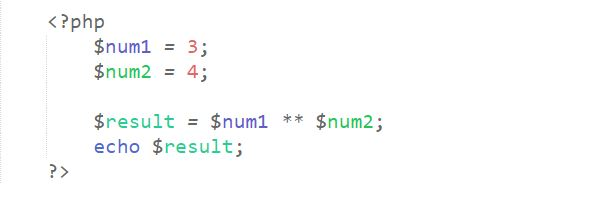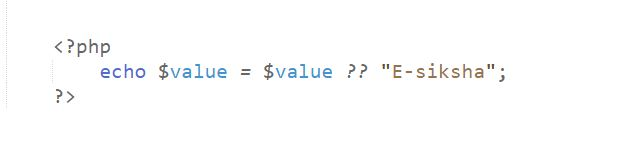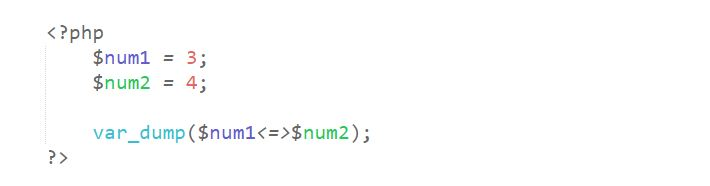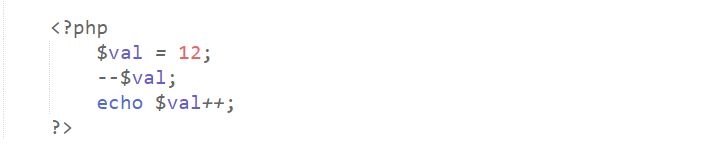# PHP Operators Quiz

Question 1.

How many basic data types are offered by PHP?

• A) 5
• B) 6
• C) 7
• D) 8
Question 2.

Which of the following operator is not available in PHP?

• A) Dot (.)
• B) =
• C) .=
• D) @
Question 3.

Which of the following operator is known as null coalescing in PHP?

• A) < == >
• B) ===
• C) ??
• D) ?
Question 4.

The "**" operator belongs to which category of operators?

• A) Arithmetic Operator
• B) Logical Operator
• C) Comparison Operator
• D) Conditional Operator
Question 5.

What is the correct output of the given code snippets?• A) 12
• B) 81
• C) 64
• D) Garbage Value
Question 6.

Which logical operator has the higher precedence from the following?

• A) &
• B) !
• C) ||
• D) xor
Question 7.

What is the correct output of given code snippets?• A) E-siksha
• B) Garbage Value
• C) Error
• D) None of the Above.
Question 8.

What is the correct output of given code snippets?• A) int(0)
• B) int(-1)
• C) int(1)
• D) Error
Question 9.

How would you increase \$num by 5 using an assignment operator?

• A) \$num = \$num + 5;
• B) \$num = \$num + \$num + \$num + \$num + \$num;
• C) \$num += 5;
• D) None of the Above.
Question 10.

What will be the output of the following PHP code?• A) 11
• B) 12
• C) 10
• D) Error
Recent Articles